# Texas Go Math Grade 3 Lesson 4.1 Answer Key Round to the Nearest Ten or Hundred

Refer to our Texas Go Math Grade 3 Answer Key Pdf to score good marks in the exams. Test yourself by practicing the problems from Texas Go Math Grade 3 Lesson 4.1 Answer Key Round to the Nearest Ten or Hundred.

## Texas Go Math Grade 3 Lesson 4.1 Answer Key Round to the Nearest Ten or Hundred

Unlock the Problem

Mia’s baseball bat is 32 inches long. What is its length rounded to the nearest ten inches?
30 inches
Explanation:
To round a two-digit number to the nearest ten,
simply increase it or decrease it to the nearest number that ends in 0.
When a number ends in 1, 2, 3, or 4, bring it down;
in other words, keep the tens digit the same and turn the ones digit into a 0.

Remember
When you round a number you find a number that tells you about how much or about how many.
When rounding a number you will “round up” or “round down”.
When the number you are rounding is between 0-4, you round down to the next lowest number.
When the number is 5-9, you round the number up to the next highest number.
When we round a number, we round it to the nearest place value.

One Way Use place value to round.

A. Round 32 to the nearest ten.
Think: The digit in the ones place tells if the number is closer to 30 or 40.2 < 5
So, the tens digit stays the same. Write 3 as the tens digit.
Write zero as the ones digit.
So, the length of Mias bat rounded to the nearest ten inches is ________ inches.
30 inches
Explanation:
Mia’s baseball bat is 32 inches long, which is given in the above question.
When we round the length of Mias bat to the nearest ten inches it becomes 30 inches.

B. Round 457 to the nearest hundred.Think: The digit in the tens place tells if the number is closer to 400 or 500.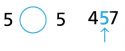5 = 5
So, the hundreds digit increases by one.
Write 5 as the hundreds digit.
Write zeros as the tens and ones digits.
So, 457 rounded to the nearest hundred is ________.
Explanation:
To round a number to the nearest 100, look at the tens digit at first.
If the tens digit is 5 or more, round up.
If the tens digit is 4 or less, round down.

Another Way Use a number line to round.

Locate and label 718 on the number lines.

A. Round 718 to the nearest ten.718 is closer to ________ than it is to ________.
So, 718 rounds to ________.
Explanation:
718 is closer to 720 than it is to 710.
So, 718 rounds to 720.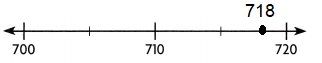B. Round 718 to the nearest hundred.718 is closer to ________ than it is to ________.
So. 718 rounds to ________.
Explanation:718 is closer to 700 than it is to 750.
So. 718 rounds to 700.

Math Talk
Mathematical Processes

What is the greatest number that rounds to 50 when rounded to the nearest ten? What is the least number? Explain.
Greatest number is 53
Least number is 49
Explanation:
53 is situated between 50 and 60.
53 is before 55, so it is rounded down to 50.
48 is between 40 and 50 and rounded to the nearest 10 is 50

Share and Show

Locate and label 46 on the number line. Round to the nearest ten.Question 1.
46 is closer to _______ than it is to __________.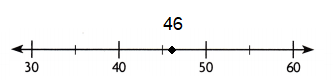46 is closer to 50 than it is to 50.
Explanation:
46 is between 40 and 50 and rounded to the nearest 10 is 50.

Question 2.
46 rounded to the nearest ten is __________.
Explanation:
46 rounded to the nearest ten is 50, as it lies between 45 and 50

Round to the nearest ten.

Question 3.
51 __________
Explanation:
51 rounded to the nearest ten is 50, as it lies between 50 and 55.

Round to the nearest hundred.

Question 4.
463 __________
Explanation:
To round a number to the nearest 100, look at the tens digit at first.
If the tens digit is 5 or more, round up.
463 rounded to the nearest hundred is 500.

Round to the nearest ten and hundred.

Question 5.
576
576 rounded to the nearest ten is 580
576 rounded to the nearest hundred is 600
Explanation:
To round a number to the nearest 100, look at the tens digit at first.
If the tens digit is 5 or more, round up.
To round a two-digit number to the nearest ten,
simply increase it or decrease it to the nearest number that ends in 0.

Question 6.
298
298 rounded to the nearest ten is 300
298 rounded to the nearest hundred is 300
Explanation:
To round a number to the nearest 100, look at the tens digit at first.
If the tens digit is 5 or more, round up.
To round a two-digit number to the nearest ten,
simply increase it or decrease it to the nearest number that ends in 0.

Question 7.
844
844 rounded to the nearest ten is 840
844 rounded to the nearest hundred is 800
Explanation:
To round a number to the nearest 100, look at the tens digit at first.
If the tens digit is 5 or more, round up.
To round a two-digit number to the nearest ten,
simply increase it or decrease it to the nearest number that ends in 0.

Problem Solving

Use the table for 8-11.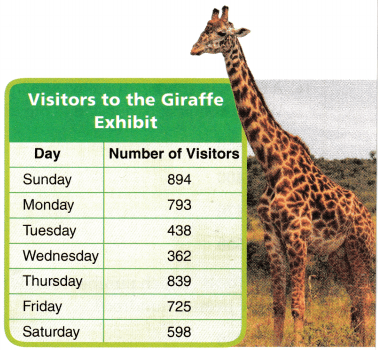Question 8.
On which day did about 900 visitors come to the giraffe exhibit?
Sunday
894 is rounded to 900, nearest hundred
Explanation:
When we observe the table of visitors come to the giraffe exhibit,
the visitors on sun day are more.
Question 9.
To the nearest ten, how many visitors came to the giraffe exhibit on Sunday?
Explanation:
To round a number to the nearest 10, look at the ones digit at first.
If the ones digit is 5 or more, round up.
as 894 is below 895, rounding the 894 to 890

Question 10.
To the nearest hundred, how many visitors came to the giraffe exhibit on Tuesday ?
Explanation:
To round a number to the nearest 100, look at the tens digit at first.
If the tens digit is 5 or more, round up.
438 is nearer to 400, to the nearest hundred.

Question 11.
Multi-Step On which two days did about 800 visitors come to the giraffe exhibit?
793 and 839 are nearer to 800, rounding to to the hundred

Question 12.
H.O.T. Write five numbers that round to 360 when rounded to the nearest ten.355, 356, 357, 358, 359, 361, 362, 363, 364 are the numbers rounded to the nearest ten 360.
Explanation:
Explanation:
To round a number to the nearest 10, look at the oness digit at first.
If the ones digit is 5 or more, round up.
If the ones digit is 5 or less, round up.

Question 13.
H.O.T. What’s the Error? Cole said that 555 rounded to the nearest ten is 600. What is Cole’s error? Explain.
Explanation:
When 555 is rounded to 560, to the nearest ten.
But Cole rounded to the nearest hundreds i.e., is 600.

Fill in the bubble for the correct answer choice.

Question 14.
A fountain shoots water 460 feet into the air. Which shows 460 rounded to the nearest hundred?
(A) 450
(B) 400
(C) 470
(D) 500
Option (D)
Explanation:
A fountain shoots water 460 feet into the air.
When 460 rounded to the nearest hundred, we get 500.

Question 15.
Use Tools Which number rounds to 300 when rounded to the nearest hundred?
(A) 380
(B) 328
(C) 249
(D) 360
Option (B)
Explanation:
To round a number to the nearest 100, look at the tens digit at first.
If the tens digit is 5 or more, round up to nearest hundred.
328 will be rounded to 300.

Question 16.
Multi-Step A train has four cars. There are 29 people in the first car and 39 people in the second car. There are 33 people in the third car and 42 people in the fourth car. Which is closest to the total number of people in the second and third car?
(A) 70
(B) 50
(C) 80
(D) 90
Option (A)
Explanation:
There are 29 people in the first car.
There are 39 people in the second car.
There are 33 people in the third car.
There are 42 people in the fourth car.
Which is closest to the total number of people in the second and third car
39 + 33 = 72
72 can be rounded to 70

Texas Test Prep

Question 17.
Use Tools What is 438 rounded to the nearest ten?
(A) 400
(B) 440
(C) 450
(D) 430
Option (B)
Explanation:
To round a number to the nearest 100, look at the tens digit at first.
If the tens digit is 5 or more, round up to nearest hundred.
438 is rounded to be 440 to the nearest ten.

### Texas Go Math Grade 3 Lesson 4.1 Homework and Practice Answer Key

Locate and label 739 on the number line. Round to the nearest hundred.Question 1.
739 is between 700 and 800.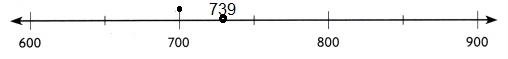Explanation:
To round a number to the nearest 100, look at the tens digit at first.
If the tens digit is 5 or less, round up to nearest hundred.
So, 739 is closest to 700.

Question 2.
739 is closer to ________ than it is to ________.
739 is closer to 700 than it is to 800.Explanation:
To round a number to the nearest 100, look at the tens digit at first.
If the tens digit is 5 or less, round up to nearest hundred.
So, 739 is nearest to 700.

Question 3.
739 rounded to the nearest hundred is ________.
739 rounded to the nearest hundred is 700.Explanation:
To round a number to the nearest 100, look at the tens digit at first.
If the tens digit is 5 or less, round up to nearest hundred.

Round to the nearest ten and hundred.

Question 4.
636
636 rounded to the nearest ten is 640
636 rounded to the nearest hundred is 600
Explanation:
To round a number to the nearest 100, look at the tens digit at first.
If the tens digit is 5 less or more, round up.
636 rounded to the nearest ten is 640, as it lies between 635 and 640.
Question 5.
215
215 rounded to the nearest ten is 220
215 rounded to the nearest hundred is 200
Explanation:
To round a number to the nearest 100, look at the tens digit at first.
If the tens digit is 5 less or more, round up.
215 rounded to the nearest ten is 220, as it lies between 215 and 220.

Question 6.
767
767 rounded to the nearest ten is 770
767 rounded to the nearest hundred is 800
Explanation:
To round a number to the nearest 100, look at the tens digit at first.
If the tens digit is 5 less or more, round up.
767 rounded to the nearest ten is 770, as it lies between 765 and 770.

Question 7.
198
198 rounded to the nearest ten is 200
198 rounded to the nearest hundred is 200
Explanation:
To round a number to the nearest 100, look at the tens digit at first.
If the tens digit is 5 less or more, round up.
198 rounded to the nearest ten is 200, as it lies between 195 and 200.

Question 8.
547
547 rounded to the nearest ten is 550
547 rounded to the nearest hundred is 500
Explanation:
To round a number to the nearest 100, look at the tens digit at first.
If the tens digit is 5 less or more, round up.
547 rounded to the nearest ten is 550, as it lies between 545 and 550.

Question 9.
852
852 rounded to the nearest ten is 850
852 rounded to the nearest hundred is 900
Explanation:
To round a number to the nearest 100, look at the tens digit at first.
If the tens digit is 5 less or more, round up.
852 rounded to the nearest ten is 850, as it lies between 850 and 855.

Problem Solving

Question 10.
A team sweatshirt costs $38. What is this cost rounded to the nearest ten dollars? Answer: 40 dollars. Explanation: sweatshirt costs$38.
When rounded to the nearest ten dollars, it is 40.

Question 11.
There are 372 workers at a software company. What is this number rounded to the nearest hundred?
Explanation:
Number of workers at a software company is 372.
When 372 is rounded to the nearest hundred, it is 400.

Lesson Check

Question 12.
Which number rounds to 340 when rounded to the nearest ten?
(A) 345
(B) 334
(C) 335
(D) 346
Option (C)
Explanation:
335 and above 335 will be rounded to 340

Question 13.
Which number rounds to 500 when rounded to the nearest hundred?
(A) 552
(B) 548
(C) 446
(D) 560
Option (B)
Explanation:
549 and below numbers can be rounded to 500

Use the table for 14-16.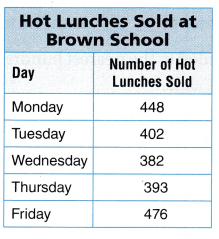Question 14.
Which is the number of hot lunches sold on Monday rounded to the nearest ten?
(A) 400
(B) 450
(C) 500
(D) 440
Option (B)
Explanation:
In the above given table number of hot lunches sold on Monday are 448.
448 and below numbers can be rounded to 450 to the nearest ten.

Question 15.
Multi-Step Which is closest to the total number of hot lunches sold on Thursday and Friday?
(A) 400
(B) 800
(C) 500
(D) 900
Explanation:
In the above given table,
number of hot lunches sold on Thursday are 393.
number of hot lunches sold on Friday are 476.
number of hot lunches sold together on both the days are
393 + 476 = 869
869 can be rounded to 900 to the nearest hundred.

Question 16.
Multi-Step Which is closest to the difference of the number of hot lunches sold on Monday and Tuesday?
(A) 50
(B) 60
(C) 100
(D) 40